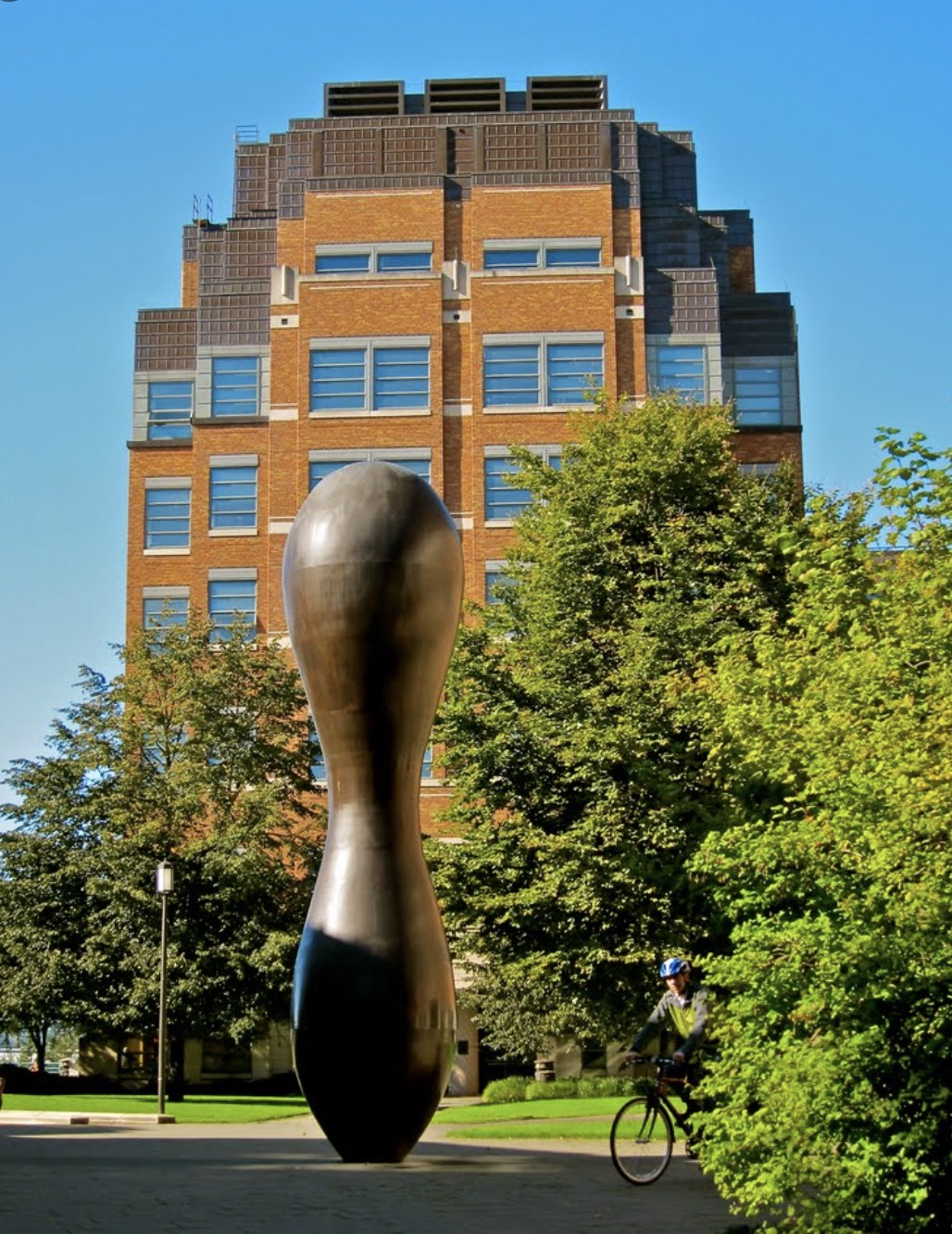# Events# Efficient Fully-Coherent Quantum Signal Processing Algorithms for Real-Time Dynamics Simulation

Yuan Liu, Massachusetts Institute of TechnologySimulating the unitary dynamics of a quantum system is a fundamental problem of quantum mechanics, in which quantum computers are believed to have significant advantage over their classical counterparts. One prominent such instance is the simulation of electronic dynamics, which plays an essential role in chemical reactions, non-equilibrium dynamics, and material design. These systems are often time-dependent, which requires that the corresponding simulation algorithm can be successfully concatenated with itself over different time intervals to reproduce the overall coherent quantum dynamics of the system. In this paper, we quantify such simulation algorithms by a property called fully-coherent: the algorithm succeeds with arbitrarily high success probability $1-\delta$, while only requiring a single copy of the initial state. We subsequently develop fully-coherent simulation algorithms based on quantum signal processing (QSP), including a novel algorithm that circumvents the use of amplitude amplification while also achieving a query complexity additive in time $t$, $\ln(1/\delta)$, and $\ln(1/\epsilon)$ for error tolerance $\epsilon$: $\Theta\big( \|\mathcal{H}\| |t| + \ln(1/\epsilon) + \ln(1/\delta)\big)$. Furthermore, we numerically analyze these algorithms by applying them to the simulation of the spin dynamics of the Heisenberg model and the correlated electronic dynamics of an H$_2$ molecule. Since any electronic Hamiltonian can be mapped to a spin Hamiltonian, our algorithm can efficiently simulate time-dependent ab initio electronic dynamics in the circuit model of quantum computation. Accordingly, it is also our hope that the present work serves as a bridge between QSP-based quantum algorithms and chemical dynamics, stimulating a cross-fertilization between these exciting fields.

Nov 02 2022
Expired!

### Time

1:00 pm - 2:00 pm### Location

UW, 15th and Pacific, Seattle
###### Website
https://phys.washington.edu/
Category